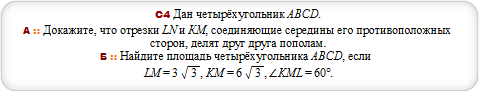# Закажи себе WolframAlpha|Pro! Получи пошаговые решения твоих задач!

## ЕГЭ с Wolfram|Alpha :: 19.05.14 :: Демон 1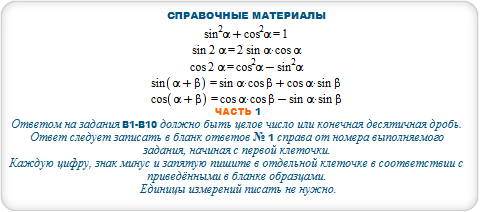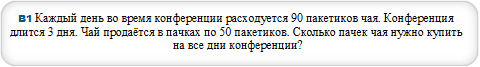#### ceil(a*b/c) for a = 90, b = 3, c=50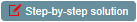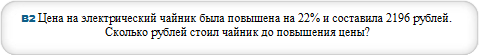#### s = n/(1+(1/100)*p) where n=2196,p=22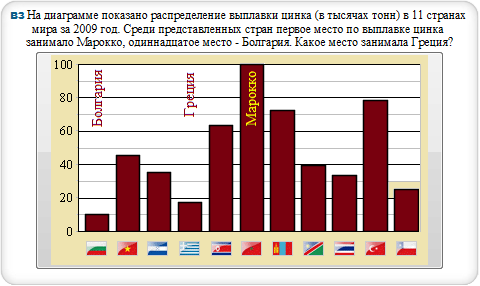#### BarChart(0, 10, 45, 35, 17, 63, 100, 72, 39, 33, 78, 25)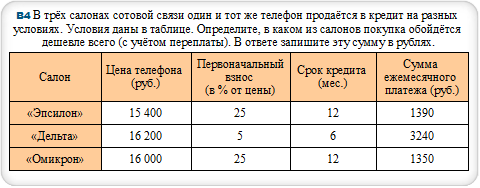#### Z = (1/100)*c*C+n*w where c=25,C=16000,w=1350,n=12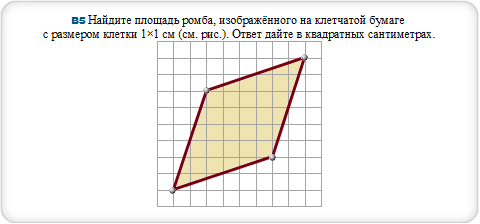#### polygon [0,0],[2,6],[8,8],[6,2]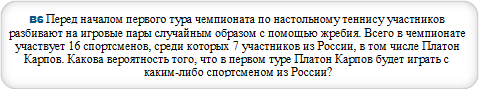#### p=(m-1)/(n-1) where m=7,n=16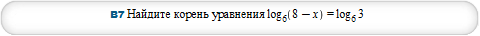#### log(6,8-x)=log(6,3)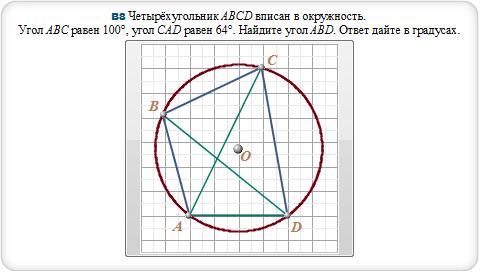#### polygon [-3.1,4.1],[2,0],[.9,6],[-2,0],[-3.1,4.1],[.9,6] and circle [-2,0],[-3.1,4.1],[.9,6]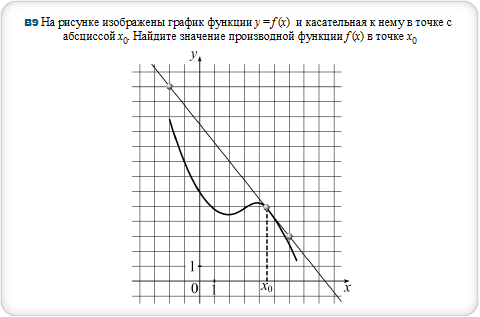#### fit polynomial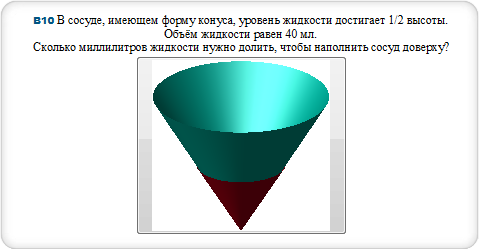#### cone with radius 2 and height 4, red cone with radius 1 and height 2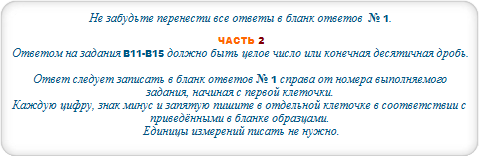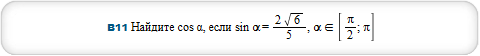#### cos(Pi-arcsin(2*sqrt(6)/5))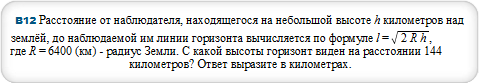#### N[l^2/(2*R)] where l=144,R=6400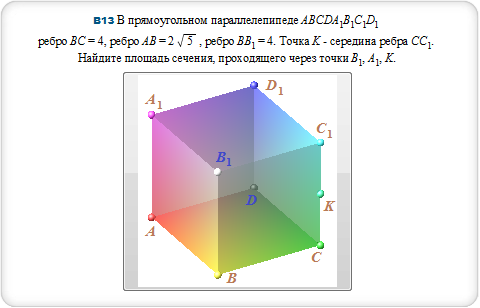#### [0,0,2],[4,0,4],[4,2sqrt5,4],[0,2sqrt5,2] and [4,0,0],[0,0,0],[0,2sqrt5,0],[4,2sqrt5,0]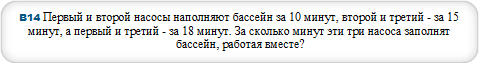#### t = 2/(1/k+1/m+1/n) for k=10,m=15,n=18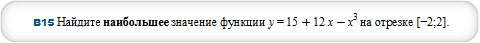#### maximize -x^3+12*x+15 over [-2,2]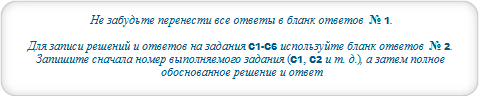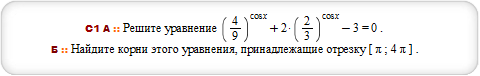#### plot (4/9)^cosx,-2*(2/3)^cosx+3 for x=Pi..4*Pi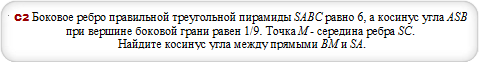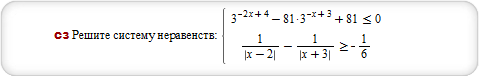#### solve {3^(-2*x+4)-81*3^(-x+3)+81 <= 0,1/abs(x-2)-1/abs(x+3) >= -1/6}